# How to Calculate and Solve for Temperature, Number of Moles, Volume, Van’t Hoff Factor and Osmotic Pressure | The Calculator Encyclopedia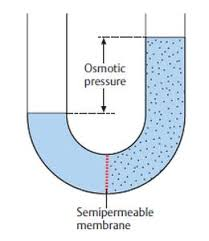The image above represents the osmotic pressure.

To compute for the osmotic pressure, five parameters are needed and these parameters are Ideal Gas Constant (R)Temperature in Kelvin (T), Number of Moles (n), Volume (V) and Van’t Hoff’s Factor (i).

The formula for calculating osmotic pressure:

π = i nRTV

Where;
π = osmotic pressure
n = number of moles
R = ideal gas constant
T = temperature in Kelvin
i = Van’t Hoff’s Factor
V = Volume

Let’s solve an example;
Find the osmotic pressure when the ideal gas constant is 0.08206 with a temperature in kelvin of 120, number of moles is 32, a volume of 48 and a van’t hoff’s factor of 24.

This implies that;
n = number of moles = 32
R = ideal gas constant = 0.08206
T = temperature in Kelvin = 120
i = Van’t Hoff’s Factor = 24
V = Volume = 48

π = i nRTV
π = 24 32 x 0.08206 x 12048
π = (24) (315.110)(48)
π = (24)(6.5647)
π = 157.5

Therefore, the osmotic pressure is 157.5 atm.

Calculating the Van’t Hoff’s Factor using the Osmotic Pressure, Number of Moles, Temperature in Kelvin, Ideal Gas Constant and Volume.

i = / nRT

Where;
i = Van’t Hoff’s Factor
π = osmotic pressure
V = Volume
n = number of moles
R = ideal gas constant
T = temperature in Kelvin

Let’s solve an example;
Find the Van’t Hoff’s Factor when the osmotic pressure is 220, volume of 50, temperature in kelvin of 180 and number of moles of 60. (R = 0.08206)

This implies that;
π = osmotic pressure = 220
V = Volume = 50
n = number of moles = 60
R = ideal gas constant = 0.08206
T = temperature in Kelvin = 180

i = / nRT
i = 50 x 220 / 60 x 0.08206 x 180
i = 11000 / 866.808
i = 12.69

Therefore, the Van’t Hoff’s Factor is 12.69.

Calculating the Volume using the Osmotic Pressure, Number of Moles, Temperature in Kelvin, Ideal Gas Constant and Van’t Hoff’s Factor.

V = i (nRT) / π

Where;
V = Volume
i = Van’t Hoff’s Factor
π = osmotic pressure
n = number of moles
R = ideal gas constant
T = temperature in Kelvin

Let’s solve an example;
Find the volume when the osmotic pressure is 280, Van’t Hoff’s Factor of 40, temperature in kelvin of 90 and number of moles of 70. (R = 0.08206)

This implies that;
i = Van’t Hoff’s Factor = 40
π = osmotic pressure = 280
n = number of moles = 70
R = ideal gas constant = 0.08206
T = temperature in Kelvin = 90

V = i (nRT) / π
V = 40 (70 x 0.08206 x 90) / 280
V = 40 (516.978) / 280
V = 20679.12 / 280
V = 73.854

Therefore, the volume is 73.854.

Calculating the Number of Moles using the Osmotic Pressure, Volume, Temperature in Kelvin, Ideal Gas Constant and Van’t Hoff’s Factor.

n = / i (RT)

Where;
n = number of moles
V = Volume
π = osmotic pressure
i = Van’t Hoff’s Factor
R = ideal gas constant
T = temperature in Kelvin

Let’s solve an example;
Find the number of moles when the osmotic pressure is 140, Van’t Hoff’s Factor of 32, temperature in kelvin of 88 and volume of 65. (R = 0.08206)

This implies that;
V = Volume = 65
π = osmotic pressure = 140
i = Van’t Hoff’s Factor = 32
R = ideal gas constant = 0.08206
T = temperature in Kelvin = 88

n = / i (RT)
n = 65 x 140 / 32 (0.08206 x 88)
n = 9100 / 32 (7.22128)
n = 9100 / 231.08096
n = 39.38

Therefore, the number of moles is 39.38.

Calculating the Temperature in Kelvin using the Osmotic Pressure, Volume, Ideal Gas Constant, Number of Moles and Van’t Hoff’s Factor.

T = / i (nR)

Where;
T = temperature in Kelvin
V = Volume
π = osmotic pressure
i = Van’t Hoff’s Factor
n = number of moles
R = ideal gas constant

Let’s solve an example;
Given that osmotic pressure is 90, Van’t Hoff’s Factor of 22, number of moles of 12 and volume of 60. (R = 0.08206). Find the temperature in kelvin?

This implies that;
V = Volume = 60
π = osmotic pressure = 90
i = Van’t Hoff’s Factor = 22
n = number of moles = 12
R = ideal gas constant = 0.08206

T = / i (nR)
T = 60 x 90 / 22 (12 x 0.08206)
T = 5400 / 22 (0.98472)
T = 5400 / 21.66384
T = 249.26

Therefore, the temperature in kelvin is 249.26.

Nickzom Calculator – The Calculator Encyclopedia is capable of calculating the osmotic pressure.

To get the answer and workings of the osmotic pressure using the Nickzom Calculator – The Calculator Encyclopedia. First, you need to obtain the app.

You can get this app via any of these means:

To get access to the professional version via web, you need to register and subscribe for NGN 1,500 per annum to have utter access to all functionalities.
You can also try the demo version via https://www.nickzom.org/calculator

Once, you have obtained the calculator encyclopedia app, proceed to the Calculator Map, then click on Basic Chemistry under Chemistry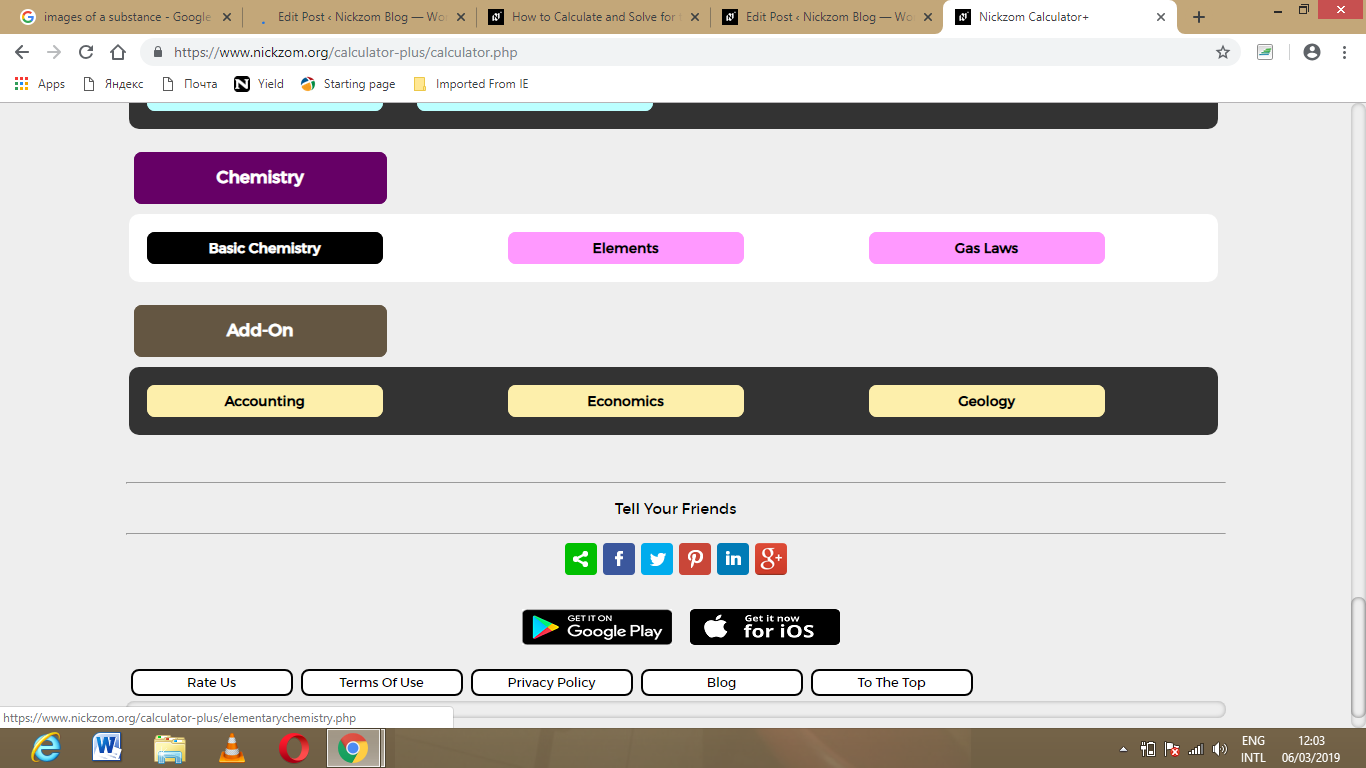Now, Click on Osmotic Pressure under Basic Chemistry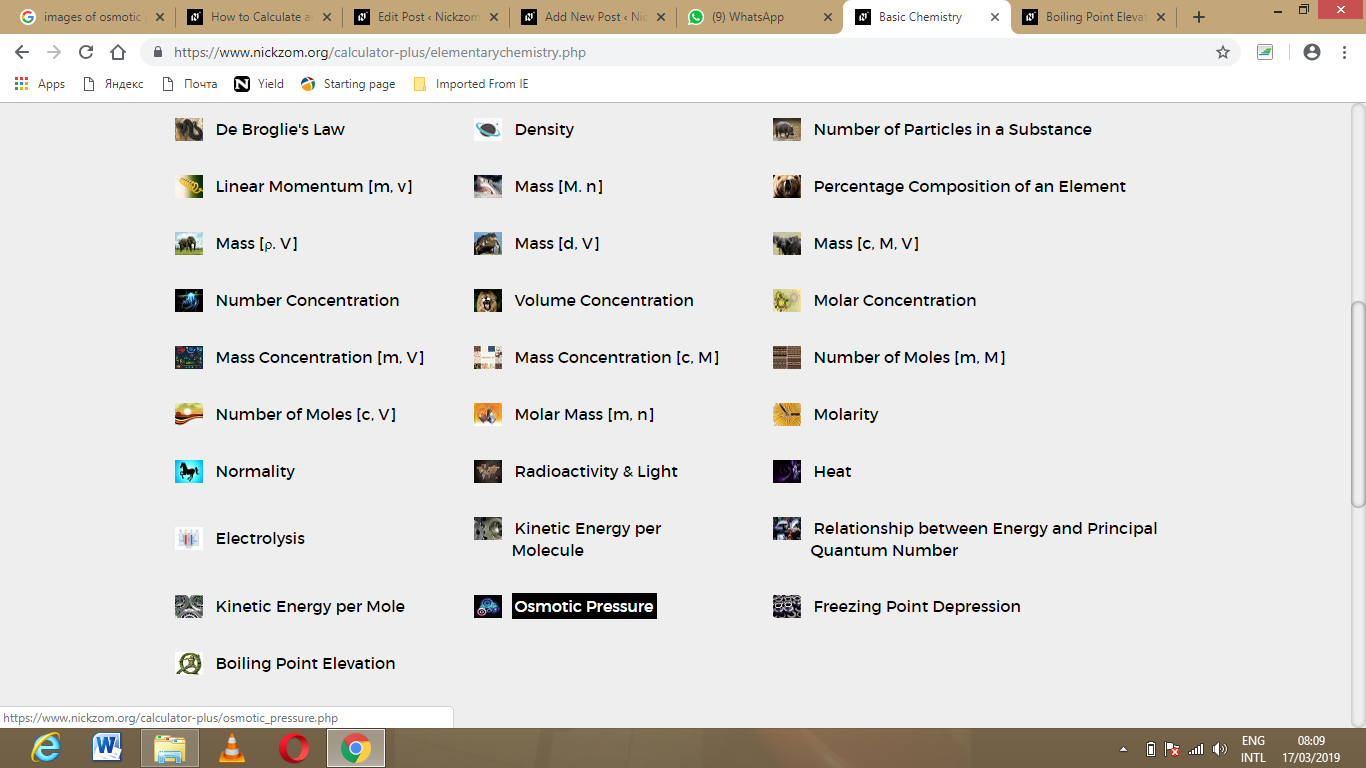The screenshot below displays the page or activity to enter your values, to get the answer for the osmotic pressure according to the respective parameters which are the Van’t Hoff’s Factor (i), Ideal Gas Constant (R), Temperature in Kelvin (T), Number of Moles (n) and Volume (V).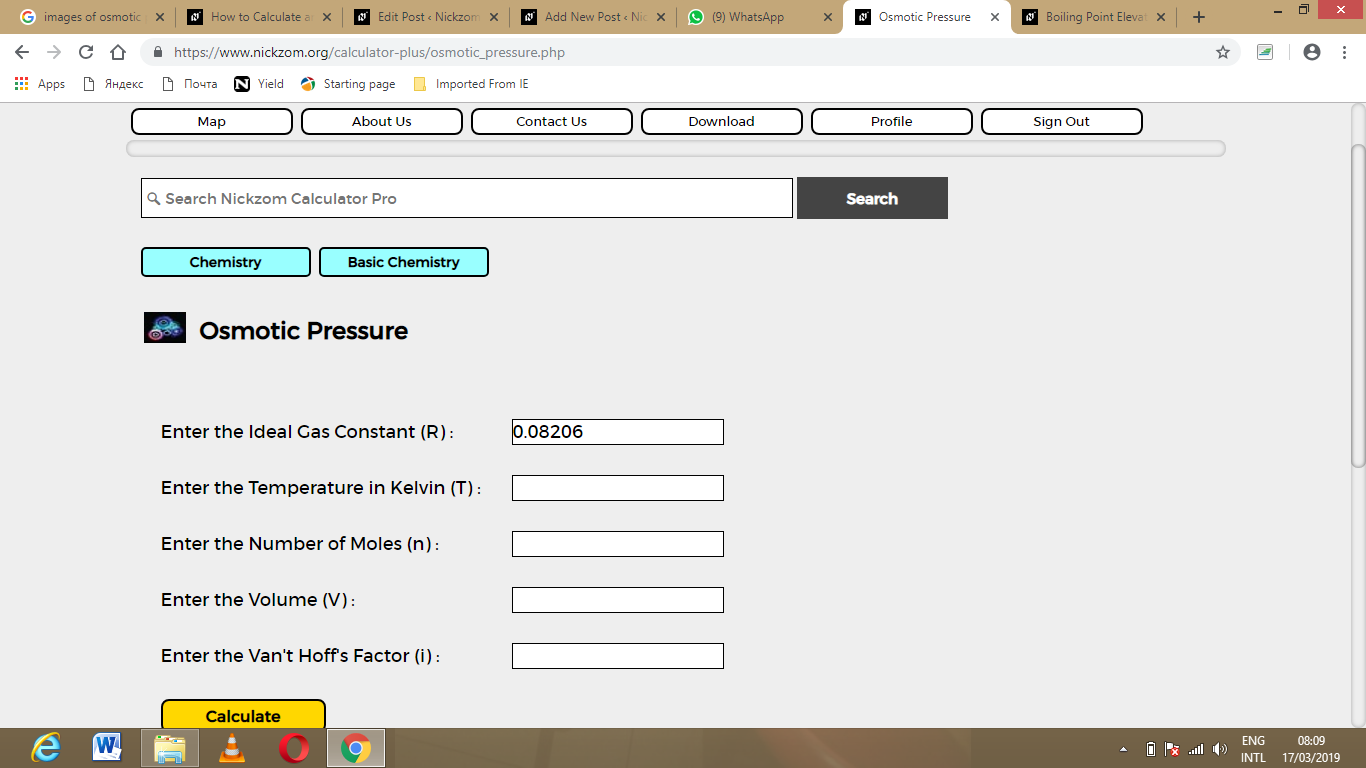Now, enter the values appropriately and accordingly for the parameters as required by the example above where the Van’t Hoff’s Factor (i) is 24, Ideal Gas Constant (R) is 0.08206, Temperature in Kelvin (T) is 120, Number of Moles (n) is 32 and Volume (V) is 48.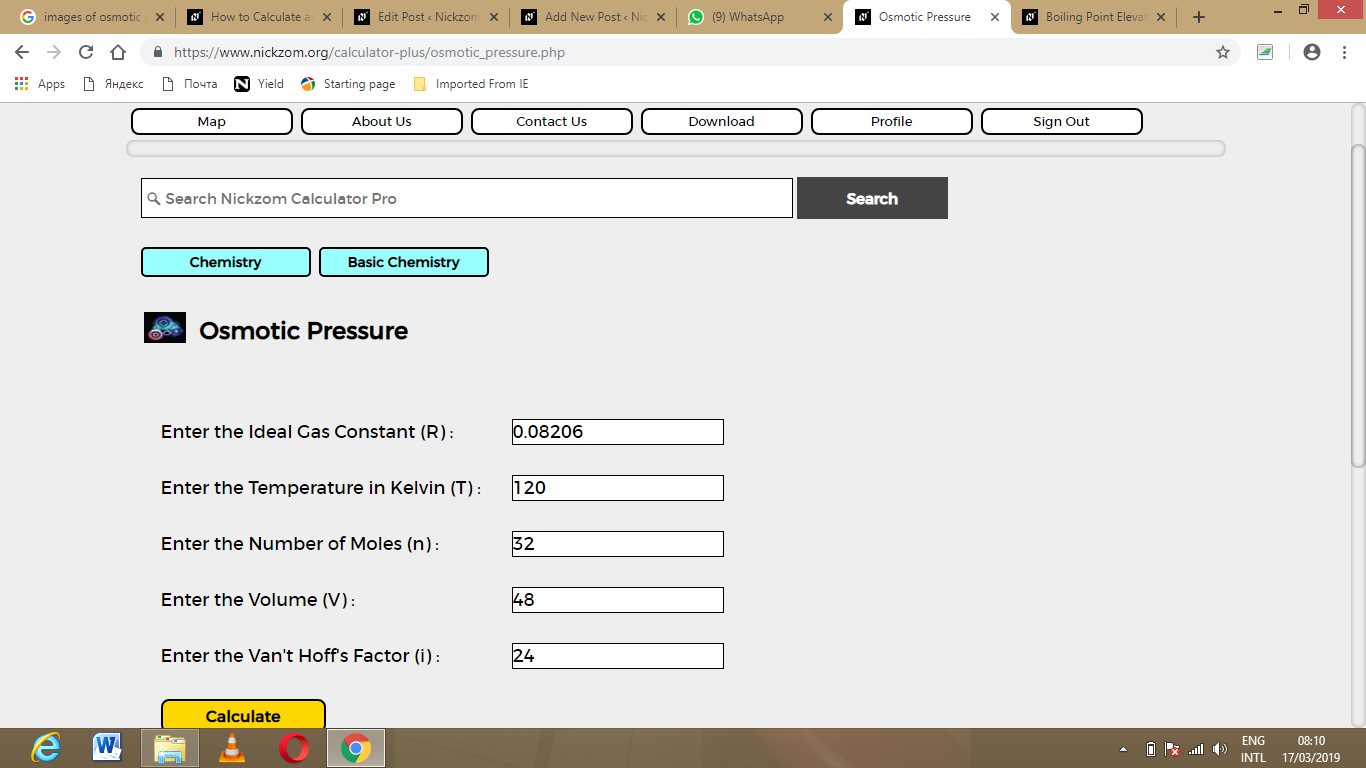Finally, Click on Calculate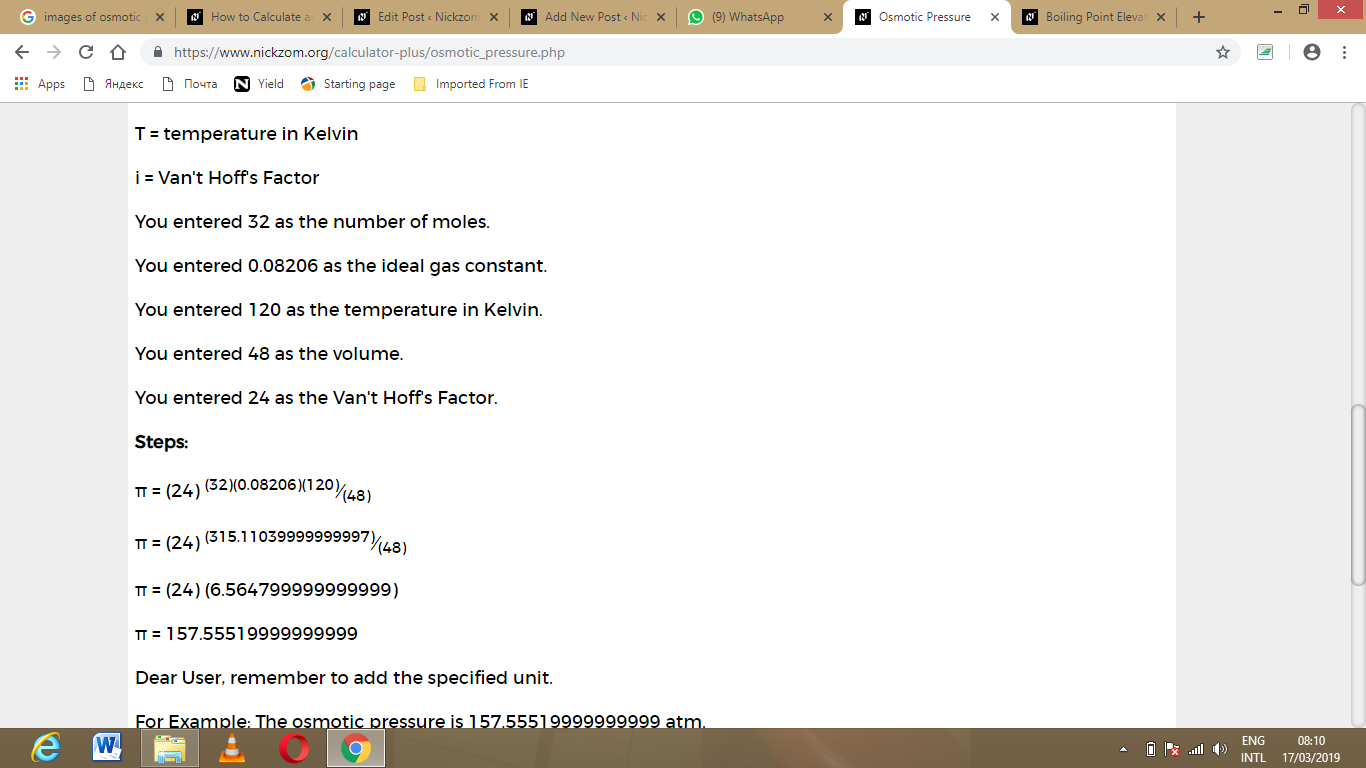As you can see from the screenshot above, Nickzom Calculator – The Calculator Encyclopedia solves for the osmotic pressure and presents the formula, workings and steps too.